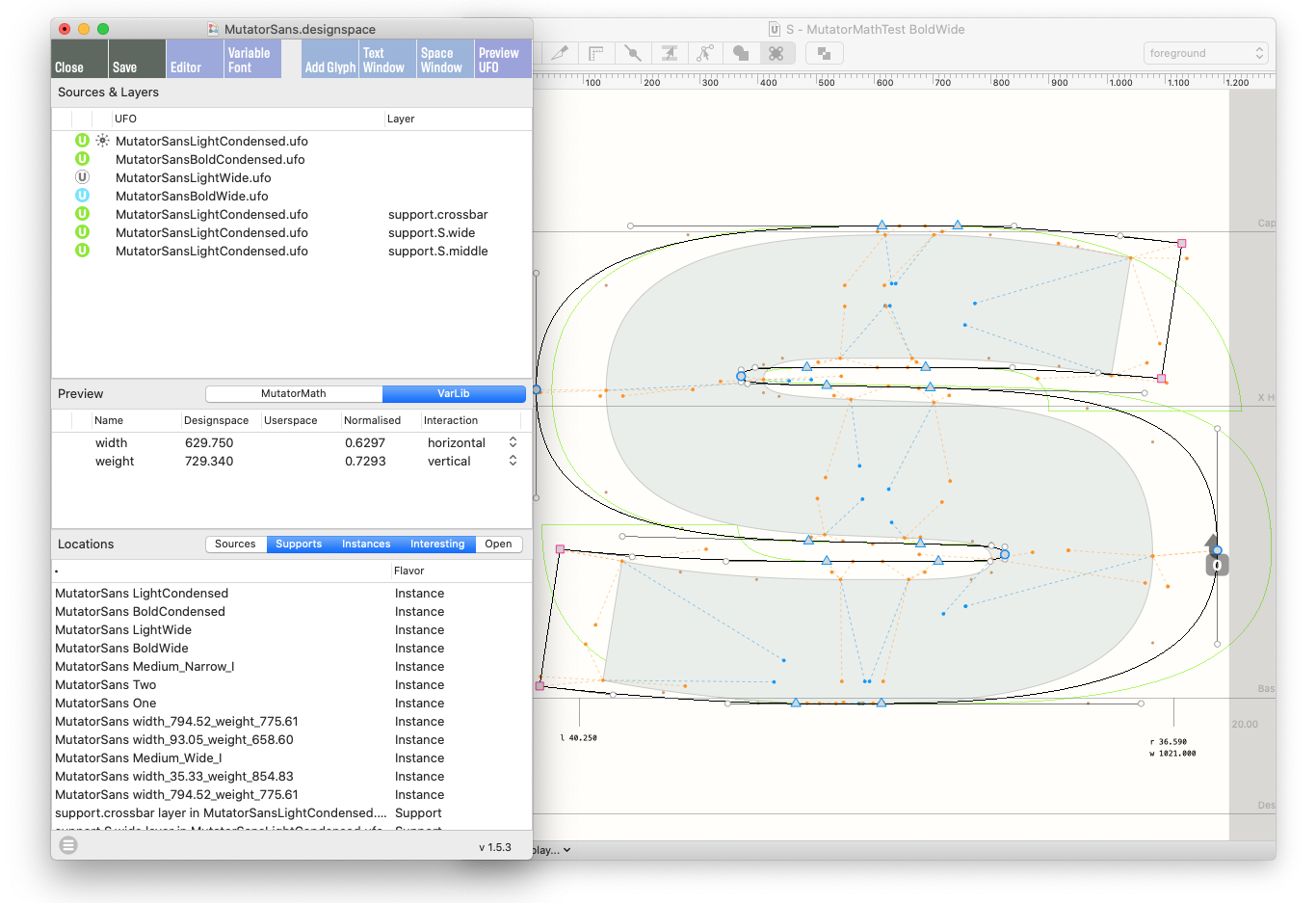## What is interpolation?

At its most basic level, interpolation is finding a number between two other numbers.

The basic interpolation formula is: start with one value (a), than add a fraction (factor) of the difference with another value (b).

``````a + factor * (b - a)
``````

Translating the formula into code:

``````def interpolateNumbers(factor, a, b):
return a + factor * (b - a)

print(interpolateNumbers(0.3, 0, 100))
print(interpolateNumbers(0.3, 200, 500))
``````
``````30.0
290.0
``````

Interpolation can be applied to anything that can be represented as numbers: position, dimensions, colors… and glyph shapes too.

## Terminology

sources
Sources of data that are fed into the interpolation function.
instances
New data produced by interpolating the sources at a certain factor.
interpolation factor

A number between `0` and `1`. For example:

• `0.0` → result is identical to the first source
• `0.5` → result is exactly between the two sources
• `1.0` → result is identical to the second source
extrapolation
Interpolation with factors outside the `0``1` range.
axis
The range of possible variation when interpolating from one source to another.

### Extrapolation

Extrapolation is using interpolation to find a number beyond the sources. This is done by using an interpolation factor that is less than `0` or greater than `1`.

``````print(interpolateNumbers(1.2, 200, 500))
print(interpolateNumbers(-0.2, 200, 500))
``````
``````560.0
140.0
``````

### Interpolating colors

To interpolate between two n-dimensional objects, we simply interpolate each dimension separately.

Here’s an example using `(r,g,b)` tuples representing colors:

``````def interpolateColors(factor, c1, c2):
# unpack color tuples
r1, g1, b1 = c1
r2, g2, b2 = c2
# interpolate each channel separately
r = interpolateNumbers(factor, r1, r2)
g = interpolateNumbers(factor, g1, g2)
b = interpolateNumbers(factor, b1, b2)
# return resulting color
return r, g, b

print(interpolateColors(0.5, (1, 0.1, 0), (1, 0, 1)))
``````
``````(1.0, 0.05, 0.5)
``````

## Interpolation requirements

Interpolation works only if the two sources have the same “topology”:

• the same number of dimensions
• matching types of dimensions

### Interpolating glyphs

A glyph is described by numbers too: the position of all points, anchors and components, the glyph’s advance width, its mark color, etc.

The RGlyph object in FontPartsAn application-independent font object API for creating and editing fonts. has an `.interpolate()` method which takes an interpolation factor and two glyphs as input:

``````glyph.interpolate(factor, glyph1, glyph2)
``````

The interpolation factor can be a tuple of two values, one for each dimension:

``````glyph.interpolate((factorX, factorY), glyph1, glyph2)
``````

The `RFont` and `RKerning` objects also have `.interpolate()` methods.

## Interpolation workflow

Interpolation can be used in different stages of a project:

### Interpolating glyphs

In the design stage, you might want to interpolate a few glyphs only, to see how the result looks like – making quick tests with key glyphs to find the right interpolation factors.

### Interpolating fonts

In the production stage, you can interpolate a whole font, or a series of fonts at once – without using the UI to speed things up.

Proper interpolation between fonts involves interpolating not just the glyphs, but also the kerning and some numerical font info attributes, such as blue zones, OS/2 weight numbers, etc.

## GlyphMath

If two glyphs are compatible, they can also be used in GlyphMath expressions.

GlyphMath uses operator overloading to add basic arithmetic operations to glyph objects: glyphs can be added or subtracted by each other, and can be multiplied or divided by a number.

GlyphMath can be used to create interpolation effects, transplant transformations from one glyph to another and superimpose several effects at once.

## MutatorMath

MutatorMath is a Python library to calculate interpolations between multiple sources in multiple dimensions. It was developed for interpolating data related to fonts, but it can handle any arithmetic object.

## Skateboard

Skateboard is a RoboFont plugin that helps you navigate and visualize designspaces right in your glyph editor. It reads designspace file and it can interpolate with MutatorMath and FontTools varLib.## Superpolator

Superpolator is a macOS application for creating font families using multidimensional interpolation. It uses MutatorMath as its interpolation engine, and offers a rich interface for creating and visualizing the interpolation space and the instances.

Last edited on 01/09/2021